GET THE APP

Power Divider with the Combination of Bagley Polygon Power Divider and Equal Split Wilkinson Power Divider | OMICS International
International Journal of Advance Innovations, Thoughts & Ideas

# Power Divider with the Combination of Bagley Polygon Power Divider and Equal Split Wilkinson Power Divider

Govind Singh Thakur*, Prashant Gupta, P. K. Singhal and Vandana Vikas Thakare

Department of electronics, Madhav Institute of Technology and Science Gwalior MP, India

*Corresponding Author:
Govind Singh Thakur
Department of electronics, Madhav Institute of Technology and Science Gwalior MP, India
E-mail: [email protected]

Visit for more related articles at International Journal of Advance Innovations, Thoughts & Ideas

#### Abstract

A 6-way power divider with the combination of Bagley polygon power divider, Wilkinson power divider and defected ground structure are proposed in this specific work. A 100 ohm isolation resistor is used to achieve good isolation in Wilkinson power divider. The proposed configuration having, 2-section of 3-way triangular shape Bagley polygon power divider connected with the output of 2-way Wilkinson power divider. Parameters of the proposed configuration are calculated at the centre frequency of 1.5 GHz. For verification the proposed design is fabricated with the dielectric constant of 4.4 and loss tangent of 0.02. The result is having triple frequency response at 1GHz, 1.5GHz and 2GHz frequency with a gap of 0.5GHz. Return loss at all the ports below -10 dB, transmission coefficient above -7.5 dB and isolation among output ports are better than -10 dB is achieved. Measured results by spectrum analyzer are compared with simulated results by computer simulation technology microwave studio and found satisfactory.

#### Keywords

Bagley polygon power divider, computer simulation technology microwave studio, defected ground structure, SMA (submarine version A) connector, spectrum analyzer, Wilkinson power divider.

#### Introduction

Power divider and combiner are very important in the microwave and millimetre wave systems. It is used to distribute input power to more than two output ports. They are having application in the field of antenna, amplifier, wireless communication system, mixer, phase shifter and measurement systems. In these dividers Wilkinson power divider, Gysel power divider and Bagley power divider are most important topic for research in recent years . An N-way Wilkinson power divider is of two types, equal split and unequal split depend on power level at the output. The original Wilkinson power divider developed by Wilkinson, consist of two quarter wavelength line and uses isolation resistor at the output . Compared to other power divider Bagley polygon power divider did not uses any isolation register, and can be easily extended to any number of output ports . In the work dual band, multi band Wilkinson power dividers [4-6], Bagley polygon power divider  has been considered.

In the proposed design a triple frequency power divider is made with the help of 2-way equal split Wilkinson power divider, 3-way triangular shape Bagley polygon power divider and defected ground structure (DGS) (etched circle in ground plane). The proposed design is simulated, by CST microwave studio using transient solver , and tested by spectrum analyzer in frequency range 0 to 3 GHz.

#### Design Methodology

The proposed configuration of the power divider with single input port and six output port is shown in figure 1. Two section of triangular Bagley power divider with the combination of Wilkinson power divider is proposed. Single isolation register is use for the equal power division at the output as shown in figure 1. For effective study single section of 3-way triangular shape Bagley polygon power divider is proposed. It has single input and three output port and it has three arms with impedance of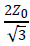ohm as shown in figure2. To accomplish the design, following electromagnetic equations used for the calculation of length and width are given belowThe empirical formula for the calculation of width to length ratio is given by equation (1)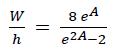(1)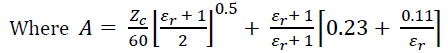(2)

Also Zc = Z0= 50 Ω, εr (dielectric constant) =4.4, W = width, h = height of dielectric that is taken as 1.6 mm with loss tangent of 0.02.

Guided Wavelength is calculated by equation (3) .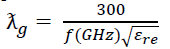(3)

Where εre = Effective dielectric constant, f = 1.5 GHz

Electrical Length is calculated by equation (4)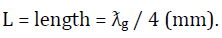(4)

Effective dielectric constant of dielectric material is given by equation (5) and equation (6) .

For W/h ≤ 1,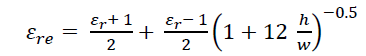(5)

For W/h>1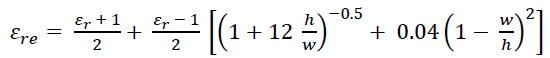(6)

Where w/h is calculated using equation (1). Values obtain after calculation is given in table 1.

#### Results

Single Section of Bagley Polygon Power Divider

Single section of Bagley power divider is test first at the centre frequency of 1.5 GHz. The return loss is measured at all ports (S11, S22, S33 and S44), by terminating all the ports except one of them with the matched load (in present case 50 ohm). The fabricated PCB (printed circuit board) Layout shown in figure 3 and Measurement setup for S11 is shown in figure 4. Return loss at port 1 (S11) works in the centre frequency of 1.5 GHz with the bandwidth of nearly 1.1 GHz Shows in figure 5. The graph ranges between -15 to -20 dB. S22, S33 and S44 are also shown in the figure 6 and figure 7, which are having return loss below - 10 dB. It shows perfect matching at all ports.

The transmission parameters of the single Section of the Bagley power divider is calculated by terminating all port except input and output port with match load. The results show proper working of the single section of the Bagley power divider at frequency of 1.5 GHz. The result having, transmission coefficient ranges between -5dB to -6dB. The graph of the transmission parameters are shown in figure 8 and figure 9.

Dual Section Bagley Power Divider with the Help of Wilkinson Power Divider

In the proposed dual section Bagley Power Divider return loss at all ports calculated as in single section Bagley power divider. The PCB Layout shown in figure 10 and measurement setup for the calculation of S11 is shown in figure 11. In case of S11 it is having triple frequency response at 1GHz, 1.5GHz, 2GHz frequency, with the return loss of -33.162dB, -23.315dB, -22.095dB respectively shown in figure 12. The proposed triple frequency configuration can be used as wide band divider in the frequency range 0.88GHz to 2.11GHz (bandwidth of 1.23 GHz). Return loss at the remaining ports ranges between -10dB to -30dB is also shown in figure 13 (S22, S33, S55 and S66) and figure 14 (S44 and S77).

The transmission parameters of dual section Bagley power divider are calculated by terminating all port except input and output port with match load (50 ohm resistance). The transmission coefficient ranges between -7dB to -8dB. Results of transmission coefficients are shown in figure 15 and figure 16.

Figure 15: Simulated and measured transmission coefficient from port1 to port 2, port 3, port 5 and 6.

#### Conclusion & Future Work

This paper presents the analysis and design of general multi frequency power divider. The proposed dual section and single section of the Bagley power divider were designed, simulated, fabricated and measured. The results of the designed power divider show the validity of the proposed design procedure and proved the multi frequency and wide band nature. Good matching at input/output ports and good isolation among output ports were achieved.

#### References

Select your language of interest to view the total content in your interested language

### Article Usage

• Total views: 13902
• [From(publication date):
May-2013 - Oct 28, 2021]
• Breakdown by view type
• HTML page views : 9765Can't read the image? click here to refresh